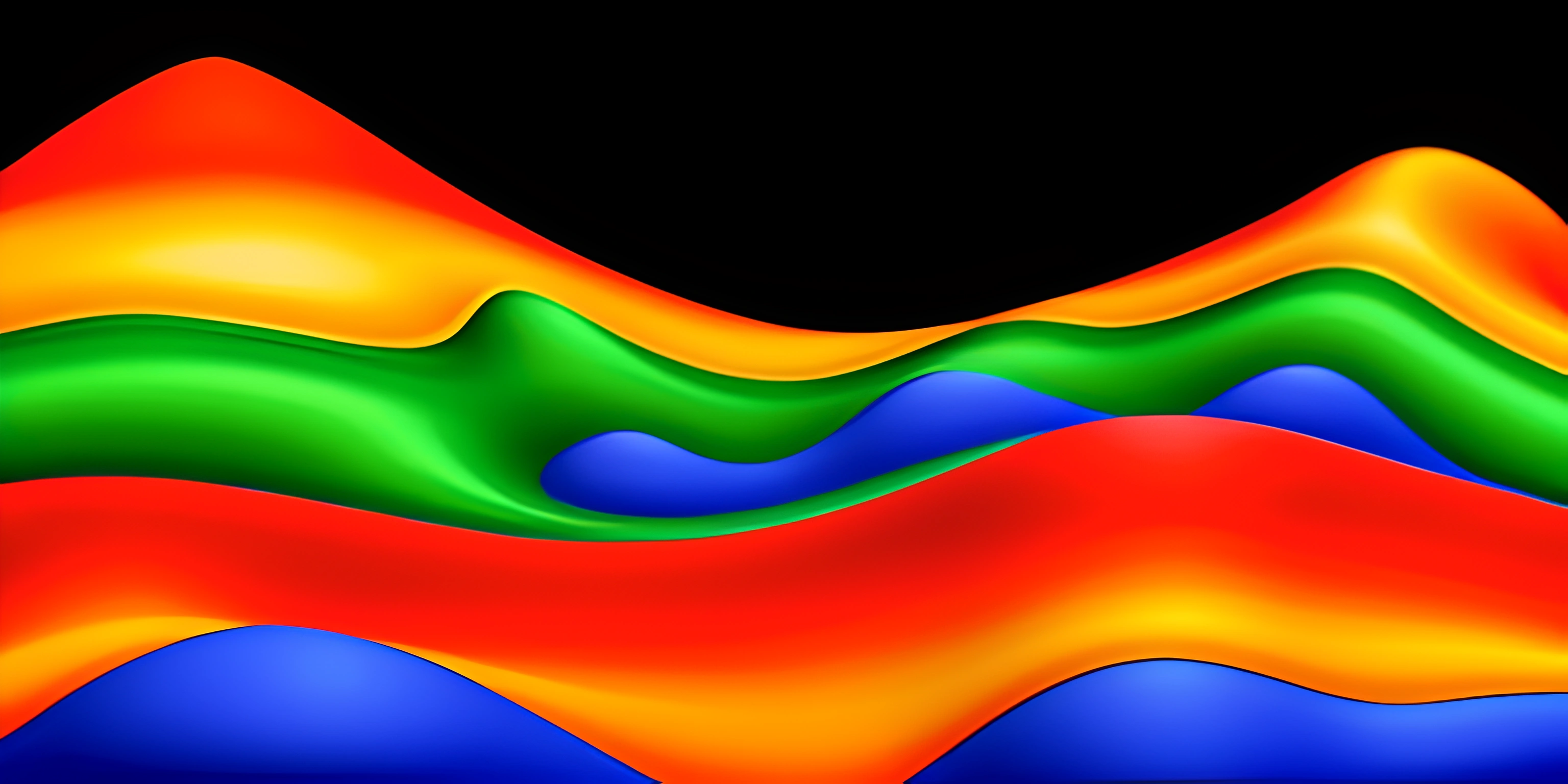# MATLAB 3D Contour Plots and Surface PlotsNote: this page has been created with the use of AI. Please take caution, and note that the content of this page does not necessarily reflect the opinion of Cratecode.

Visualizing complex data in three dimensions can be a powerful tool for understanding trends and relationships. In MATLAB, you can create 3D contour plots and surface plots to explore your data in depth. This article will guide you through the process of creating these plots and customizing their appearance.

## Create a 3D Contour Plot

To create a 3D contour plot, we will use the `contour3` function. This function requires a grid of data, which can be created using the `meshgrid` function. Here's an example of how to create a 3D contour plot:

``````% Define the function for the 3D contour plot
[X, Y] = meshgrid(-2:0.1:2, -2:0.1:2);
Z = X.^2 + Y.^2;

% Create the 3D contour plot
contour3(X, Y, Z);``````

This will create a 3D contour plot of the function Z = X^2 + Y^2.

## Create a Surface Plot

Surface plots in MATLAB can be created using the `surf` function. Like the `contour3` function, `surf` requires a grid of data points. Here's an example of creating a surface plot:

``````% Define the function for the surface plot
[X, Y] = meshgrid(-2:0.1:2, -2:0.1:2);
Z = X.^2 + Y.^2;

% Create the surface plot
surf(X, Y, Z);``````

This will produce a surface plot of the function Z = X^2 + Y^2.

## Customizing Plots

MATLAB offers numerous options for customizing the appearance of your 3D contour plots and surface plots. Here are a few examples:

### Colormaps

To change the color scheme of your plot, you can use the `colormap` function. MATLAB provides a variety of colormaps to choose from:

``````% Apply a colormap to a surface plot
surf(X, Y, Z);
colormap(jet); % Use the 'jet' colormap``````

### Axis Labels and Titles

Adding axis labels and a title to your plot can help clarify the information being displayed. Use the `xlabel`, `ylabel`, `zlabel`, and `title` functions to label your plot:

``````% Label the axes and add a title
surf(X, Y, Z);
xlabel('X-axis');
ylabel('Y-axis');
zlabel('Z-axis');
title('Surface Plot of Z = X^2 + Y^2');``````

## Conclusion

MATLAB offers powerful tools for creating 3D contour plots and surface plots, allowing you to visualize complex data in three dimensions. By customizing your plots with colormaps, axis labels, and titles, you can generate informative and visually appealing visualizations.

## FAQ

### How do you create a 3D contour plot in MATLAB?

To create a 3D contour plot in MATLAB, you'll need to use the `contour3` function along with the `meshgrid` function to create a grid of data points. For example, `[X, Y] = meshgrid(-2:0.1:2, -2:0.1:2); Z = X.^2 + Y.^2; contour3(X, Y, Z);`.

### How do you create a surface plot in MATLAB?

To create a surface plot in MATLAB, you'll need to use the `surf` function along with the `meshgrid` function to create a grid of data points. For example, `[X, Y] = meshgrid(-2:0.1:2, -2:0.1:2); Z = X.^2 + Y.^2; surf(X, Y, Z);`.

### How can you customize the appearance of 3D plots in MATLAB?

You can customize the appearance of 3D plots in MATLAB by using functions such as `colormap` to change the color scheme, and `xlabel`, `ylabel`, `zlabel`, and `title` to add labels and titles to the plot.